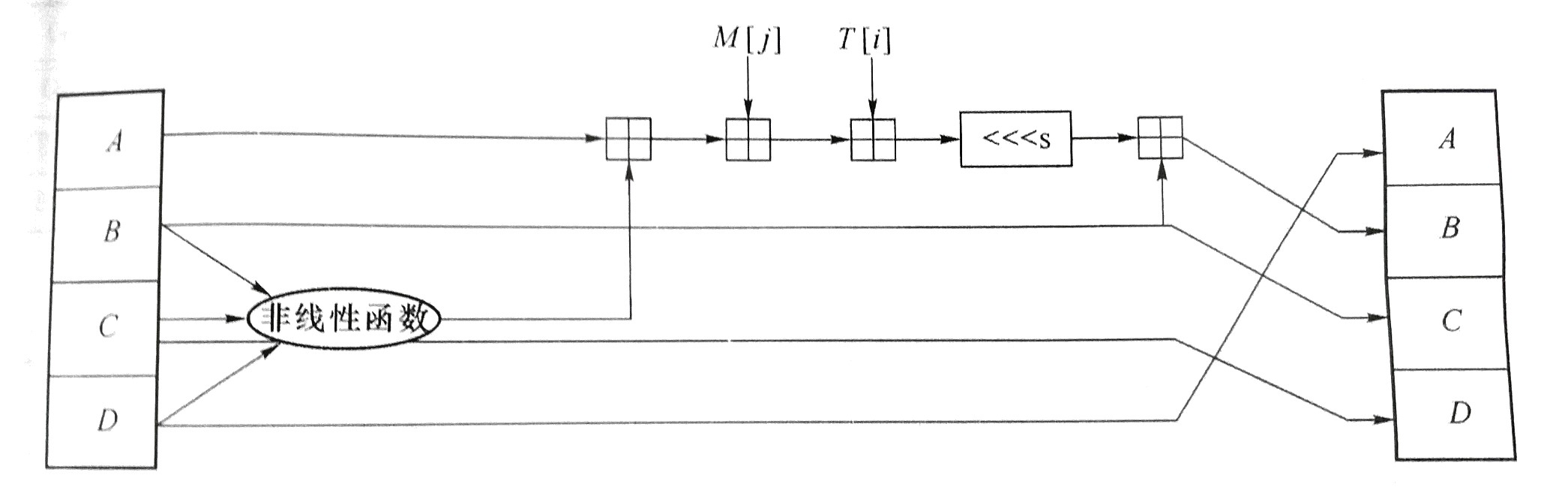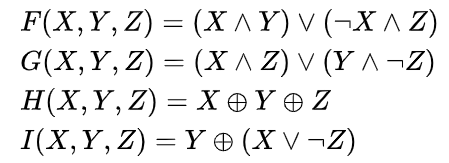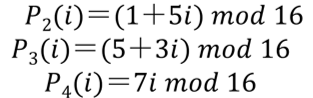【密码学】Hash函数之MD5算法source. 12月 28, 2018
• 在其它设备中阅读本文章
•Hash 函数是密码学的一个重要分支，是将任意长度的输入变换为固定长度的输出的不可逆的单向密码体制。

## 算法过程

# 将字符串转成二进制字符串
def str2Bin(m):
return "".join('{:08b}'.format(ord(x)) for x in m)
# 调用
m = str2Bin(m)


# 将大端序二进制/十六进制字符串转成小端序
# Hex == True means Hex, Hex == False means Bin
def LittleEndian(s,Hex):
l = len(s)
ans = ""
## 二进制
if Hex == False:
i = l-8
while i >= 0:
ans += s[i:i+8]
i -= 8
## 十六进制
else:
i = l-2
while i >= 0:
ans += s[i:i+2]
i -= 2
return ans


# 模2的32次方加
return (a+b)%(2**32)


### 1. 附加填充位

## Padding
lm_mod = lm%512
### 若原始消息长度刚好满足模512与448同余
### 则还需要填充512位
if lm_mod == 448:
FillLength = 512
elif lm_mod > 448:
FillLength = 512+448-lm_mod
else:
FillLength = 448-lm_mod
## 填充
m += '1'+(FillLength-1)*'0'


# Padding length
length = LittleEndian(bin(lm%(2**64))[2:].zfill(64),False)
m += length
lm = len(m)    # 填充之后的消息长度


### 2. 初始化链接变量

# Initial Variable A, B, C, D
IV_A = 0x67452301
IV_B = 0xefcdab89
IV_D = 0x10325476


### 3. 分组处理

（回想分组密码）MD5 以 512 bit 为分组长度进行分组。每个分组又被分成 16 个 32 bit 的子分组，分别参与每轮的 16 个步骤。

### 4. 步函数A 先和非线性函数的结果加一下，结果再和 M[j] 加一下，结果再和 T[i] 加一下，结果再循环左移 s 次，结果再和原来的 B 加一下，最后的得到新 B。（也是够麻烦的2333）

#### 非线性函数是啥？# Nonlinear function
F = lambda x,y,z:((x&y)|((~x)&z))
G = lambda x,y,z:((x&z)|(y&(~z)))
H = lambda x,y,z:(x^y^z)
I = lambda x,y,z:(y^(x|(~z)))


#### M[j] 是啥？# 每次 M[i]（消息分片）次序。
M = ((0,1,2,3,4,5,6,7,8,9,10,11,12,13,14,15),
(1,6,11,0,5,10,15,4,9,14,3,8,13,2,7,12),
(5,8,11,14,1,4,7,10,13,0,3,6,9,12,15,2),
(0,7,14,5,12,3,10,1,8,15,6,13,4,11,2,9))


#### 循环左移几位？

# 每次循环左移的位数
Shi = ((7,12,17,22)*4,
(5,9,14,20)*4,
(4,11,16,23)*4,
(6,10,15,21)*4)


## 完整代码

### MD5_tables.py

#-*- coding:utf-8 -*-
import math

# Initial Variable A, B, C, D
IV_A = 0x67452301
IV_B = 0xefcdab89
IV_D = 0x10325476

# Nonlinear function
F = lambda x,y,z:((x&y)|((~x)&z))
G = lambda x,y,z:((x&z)|(y&(~z)))
H = lambda x,y,z:(x^y^z)
I = lambda x,y,z:(y^(x|(~z)))
# Loop Left Shift
L = lambda x,n:(((x<<n)|(x>>(32-n)))&(0xffffffff))

# 每次循环左移的位数
Shi = ((7,12,17,22)*4,
(5,9,14,20)*4,
(4,11,16,23)*4,
(6,10,15,21)*4)

# 每次 M[i]（消息分片）次序。
M = ((0,1,2,3,4,5,6,7,8,9,10,11,12,13,14,15),
(1,6,11,0,5,10,15,4,9,14,3,8,13,2,7,12),
(5,8,11,14,1,4,7,10,13,0,3,6,9,12,15,2),
(0,7,14,5,12,3,10,1,8,15,6,13,4,11,2,9))

# 将大端序二进制/十六进制字符串转成小端序
# Hex == True means Hex, Hex == False means Bin
def LittleEndian(s,Hex):
l = len(s)
ans = ""
if Hex == False:
i = l-8
while i >= 0:
ans += s[i:i+8]
i -= 8
else:
i = l-2
while i >= 0:
ans += s[i:i+2]
i -= 2
return ans

# 将字符串转成二进制字符串
def str2Bin(m):
return "".join('{:08b}'.format(ord(x)) for x in m)

# 模2的32次方加
return (a+b)%(2**32)

# 产生常数T[i]，常数有可能超过32位，需要&0xffffffff操作
# 返回的是十进制的数。
def T(i):
result = (int(4294967296*abs(math.sin(i))))&0xffffffff
return result


### MD5.py

#-*- coding:utf-8 -*-
# Author: D. Jog Yummy
import math
from MD5_tables import *

# Calculate the MD5 value
def MD5(m):
## str to binstr
m = str2Bin(m)
lm = len(m)
lm_mod = lm%512
### 若原始消息长度刚好满足模512与448同余
### 则还需要填充512位
if lm_mod == 448:
FillLength = 512
elif lm_mod > 448:
FillLength = 512+448-lm_mod
else:
FillLength = 448-lm_mod
m += '1'+(FillLength-1)*'0'
length = LittleEndian(bin(lm%(2**64))[2:].zfill(64),False)
m += length
lm = len(m)
## Generate Initial Variable(IV)
A,B,C,D = IV_A,IV_B,IV_C,IV_D
for i in range(0,lm,512):
Y = m[i:i+512]
for j in range(4):  # 共4轮
for k in range(16): # 每轮16个步骤
# A, B, C, D Backup
AA,BB,CC,DD = A,B,C,D
## Nonlinear function
if j == 0:
t = F(B,C,D)
elif j == 1:
t = G(B,C,D)
elif j == 2:
t = H(B,C,D)
else:
t = I(B,C,D)
T_i = T(16*j+k+1)
M_j = LittleEndian(Y[M[j][k]*32:M[j][k]*32+32],False)
A, C, D = DD, BB, CC
B = L(B,Shi[j][k])
print str(16*j+k+1).zfill(2),
print hex(A).replace("0x","").replace("L","").zfill(8),
print hex(B).replace("0x","").replace("L","").zfill(8),
print hex(C).replace("0x","").replace("L","").zfill(8),
print hex(D).replace("0x","").replace("L","").zfill(8)
print "*"*38
return ans1+ans2+ans3+ans4

def main():
# m = raw_input("Please input the message: ")
m = "iscbupt"
# m = "Beijing University of Posts and Telecommunications"
ans = MD5(m)
print "The MD5 of this message is:\n\t"+MD5(m)+" (Lowercase)\n\t"+ans.upper()+" (Uppercase)"

if __name__ == '__main__':
main()